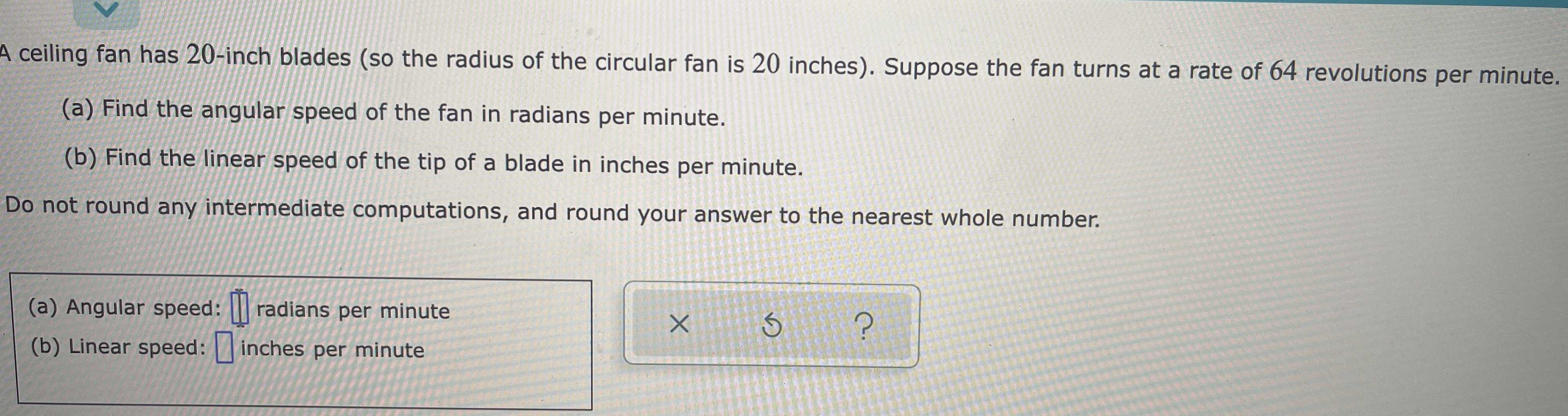### ¿Todavía tienes preguntas de matemáticas?

Pregunte a nuestros tutores expertos
Algebra
PreguntaA ceiling fan has $$20$$ -inch blades (so the radius of the circular fan is $$20$$ inches). Suppose the fan turns at a rate of $$64$$ revolutions per minute.

(a) Find the angular speed of the fan in radians per minute.

(b) Find the linear speed of the tip of a blade in inches per minute.

Do not round any intermediate computations, and round your answer to the nearest whole number.

(a) Angular speed: $$\square$$ radians per minute

(b) Linear speed: $$\square$$ inches per minute